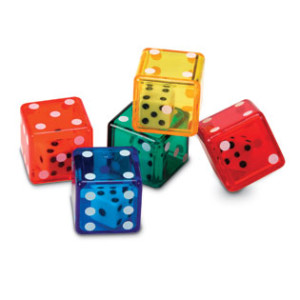The lowest unit of randomness is probably a random uniform bit, in other words a symmetric Bernoulli random variable taking the values ${0}$ and ${1}$ with probability ${1/2}$. If

$U=\sum_{n=1}^\infty b_n2^{-n}$

is the binary expansion of a random variable ${U}$ taking values in the interval ${[0,1]}$, then ${U}$ follows the uniform law on ${[0,1]}$ iff the bits ${{(b_n)}_{n\geq1}}$ are i.i.d. symmetric Bernoulli. As explained in a previous post, this observation allows for instance to extract from ${U}$ a whole infinite sequence of i.i.d. uniform random variables on ${[0,1]}$. But real real numbers are not accessible in current computers.

In a computer, everything is finite, and we use a finite sequence of bits to produce a uniform discrete random variable, up to a fixed precision, typically ${32}$ or ${64}$ bits, which corresponds to ${2^{32}}$ and ${2^{64}}$ different values respectively. More generally, the simulation of the discrete uniform law on ${n}$ different values amounts to the coding in bits of the integer ${n}$, namely ${\log_2(n)}$ bits. In particular, the simulation of a random permutation ${\sigma_n}$ on ${\{1,\ldots,n\}}$ costs approximately ${\log_2(n!)\sim n\log_2(n)}$ bits. If we use the Fisher-Yates-Knuth shuffle, we simulate ${\sigma_n}$ using the representation in terms of product of transpositions ${\sigma_n=(1,U_1)\cdots(1,U_n)}$ where ${U_1,\ldots,U_n}$ are independent with ${U_k}$ following the uniform discrete law on ${\{1,\ldots,k\}}$ for every ${1\leq k\leq n}$. Then the total cost is ${\sim\sum_{k=1}^n\log_2(k)\sim n\log_2(n)}$, as expected.

If we start from a fixed deterministic matrix ${{(x_{jk})}_{1\leq j,k\leq n}}$, and produce a random matrix

${(x_{\sigma(j,k)})}_{1\leq j,k\leq n}$

by shuffling the ${n^2}$ entries with a random uniform permutation ${\sigma}$ of ${\{1,\ldots,n^2\}}$, then we use approximately ${2n^2\log_2(n)}$ bits. On the other hand, the production of a ${n\times n}$ random matrix with i.i.d. entries following the uniform distribution on ${\{0,\ldots,N\}}$ needs ${n^2\log_2(N)}$ bits (${N=2}$ for Bernoulli entries).

This site uses Akismet to reduce spam. Learn how your comment data is processed.

Syntax · Style · .